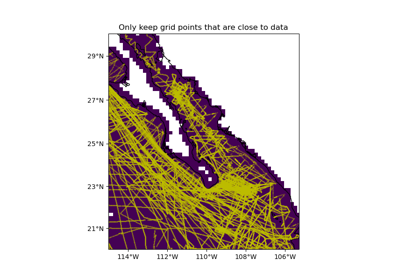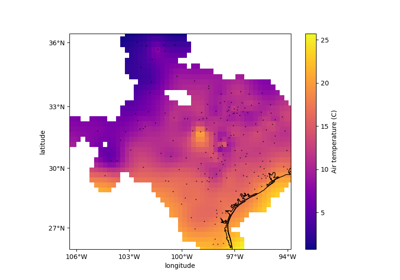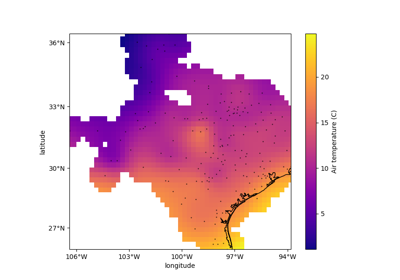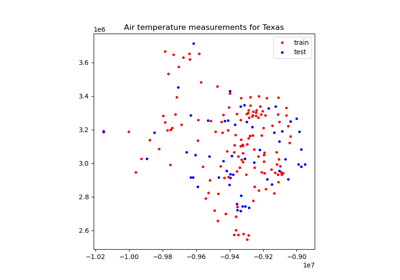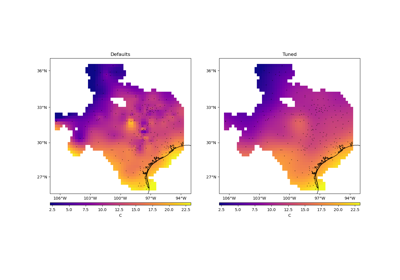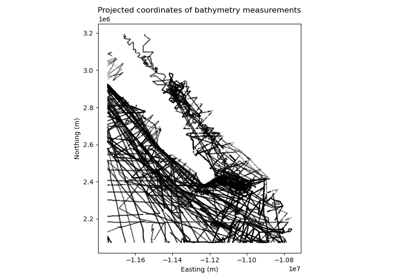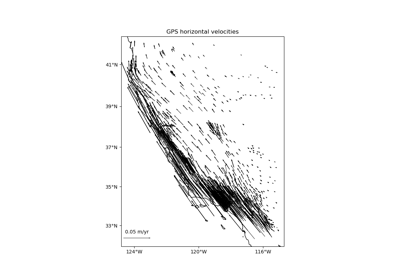## Contents

verde.distance_mask(data_coordinates, maxdist, coordinates=None, grid=None, projection=None)[source]

Mask grid points that are too far from the given data points.

Distances are Euclidean norms. If using geographic data, provide a projection function to convert coordinates to Cartesian before distance calculations.

Either coordinates or grid must be given:

• If coordinates is not None, produces an array that is False when a point is more than maxdist from the closest data point and True otherwise.

• If grid is not None, produces a mask and applies it to grid (an `xarray.Dataset`).

Note

If installed, package `pykdtree` will be used instead of `scipy.spatial.cKDTree` for better performance.

Parameters
• data_coordinates (tuple of arrays) – Same as coordinates but for the data points.

• maxdist (float) – The maximum distance that a point can be from the closest data point.

• coordinates (None or tuple of arrays) – Arrays with the coordinates of each point that will be masked. Should be in the following order: (easting, northing, …). Only easting and northing will be used, all subsequent coordinates will be ignored.

• grid (None or `xarray.Dataset`) – 2D grid with values to be masked. Will use the first two dimensions of the grid as northing and easting coordinates, respectively. For this to work, the grid dimensions must be ordered as northing then easting. The mask will be applied to grid using the `xarray.Dataset.where` method.

• projection (callable or None) – If not None, then should be a callable object ```projection(easting, northing) -> (proj_easting, proj_northing)``` that takes in easting and northing coordinate arrays and returns projected easting and northing coordinate arrays. This function will be used to project the given coordinates (or the ones extracted from the grid) before calculating distances.

Returns

mask (array or `xarray.Dataset`) – If coordinates was given, then a boolean array with the same shape as the elements of coordinates. If grid was given, then an `xarray.Dataset` with the mask applied to it.

Examples

```>>> from verde import grid_coordinates
>>> region = (0, 5, -10, -4)
>>> spacing = 1
>>> coords = grid_coordinates(region, spacing=spacing)
>>> mask = distance_mask((2.5, -7.5), maxdist=2, coordinates=coords)
[[False False False False False False]
[False False  True  True False False]
[False  True  True  True  True False]
[False  True  True  True  True False]
[False False  True  True False False]
[False False False False False False]
[False False False False False False]]
>>> # Mask an xarray.Dataset directly
>>> import xarray as xr
>>> coords_dict = {"easting": coords[0, :], "northing": coords[:, 0]}
>>> data_vars = {"scalars": (["northing", "easting"], np.ones(mask.shape))}
>>> grid = xr.Dataset(data_vars, coords=coords_dict)
>>> masked = distance_mask((3.5, -7.5), maxdist=2, grid=grid)
[[nan nan nan nan nan nan]
[nan nan nan  1.  1. nan]
[nan nan  1.  1.  1.  1.]
[nan nan  1.  1.  1.  1.]
[nan nan nan  1.  1. nan]
[nan nan nan nan nan nan]
[nan nan nan nan nan nan]]
```

## Examples using `verde.distance_mask`¶